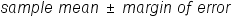+
Introduction to Confidence Intervals
Interpret Inferential Statistics
0
of 0 possible points
1 Tutorials that teach Introduction to Confidence Intervals

# Introduction to Confidence Intervals

Author: Sophia Tutorial
##### Description:

Define confidence intervals.

(more)

Sophia’s self-paced online courses are a great way to save time and money as you earn credits eligible for transfer to many different colleges and universities.*

No credit card required

37 Sophia partners guarantee credit transfer.

299 Institutions have accepted or given pre-approval for credit transfer.

* The American Council on Education's College Credit Recommendation Service (ACE Credit®) has evaluated and recommended college credit for 32 of Sophia’s online courses. Many different colleges and universities consider ACE CREDIT recommendations in determining the applicability to their course and degree programs.

Tutorial
what's covered
This lesson is about confidence levels. By the end of this lesson, you will be able to define them, and know how the work with means and how to express them. This lesson covers:
1. Confidence Intervals
2. Confidence Intervals and Means
3. Expressing Confidence Intervals

## 1. Confidence Intervals

When attempting to estimate a quantity of a population variable, you can use a single value as an estimate for that quantity. Such a value is referred to as a point estimate. Additionally, you can also provide a range to estimate such a quantity. This range is referred to as an interval estimate. In the event that you can be 100% certain that the quantity lies in this range, you can say the range is a 100% confidence interval.

However, achieving a 100% confidence interval is impossible without sampling the entire population. You can really only provide confidence intervals with levels of confidence lower than 100%. A 95% confidence interval is most commonly used. It means that researchers are 95% certain that the quantity they are estimating falls within this range. Another way to look at it is if the experiment was repeated 20 times, the population variable would fall within the 95% confidence interval in 19 of these times.

Recall from previous lessons that a population is a large group of observations, while a sample is a small group of observations drawn from a population. It is desirable to randomly select that sample to get a relatively representative portion of the population. This allows you to make more accurate predictions about the population as a whole.

## 2. Confidence Intervals and Means

The mean of a data set gives us an idea of the center of the data, regardless of whether the data set is a population or a sample. Typically, the mean of a randomly drawn sample will not be the same as the population mean. It is helpful to use a 95% confidence interval to estimate this population mean instead. Such a 95% confidence interval is equal to a sample mean plus or minus a margin of error.

formula
Confidence IntervalBy seeking the interval for a value, you are actually trying to estimate the range of values that fit within the interval you are looking at. In estimating an interval for a range of values, you must first consider a single value. Then think about how much above and how much below that specific value you are willing to look.

What if you were interested in estimating the mean hours of television that children watch per day? How would you go about estimating such a value by using sample data? You can estimate this by using a point estimate, which let’s assume in this case is 4.6 hours per day. You could also use a range to estimate this value, or an interval estimate, which maybe something along the lines of 3.8 hours per day to 5.4 hours per day.

The difference between the point estimate and the end values in the interval estimate helps you determine the margin of error you are working with. You could express the interval estimate as 4.6 hours per day, plus or minus 0.8 hours per day.

## 3. Expressing Confidence Intervals

Let’s look at estimating how much Americans spend on dining out per week. While you are interested in the overall population, you realize that asking everyone to detail their dining expenses is impossible. So you opt instead to select a random sample.

Suppose that you were to take such a sample of 500 people and determine a sample mean of \$77.48 per week was spent on dining out. This would be considered a point estimate for the mean of the overall population. Now suppose that there is a margin of error of \$10.21.

try it
Using the \$10.21 margin of error, calculate your confidence interval.

The 95% confidence interval would be calculated by subtracting \$10.21 from \$77.48, and also adding \$10.21 to \$77.48. This would give a 95% confidence interval of \$67.27 to \$87.69.

This 95% confidence interval provides you with a likely range for the population mean, which means you would be 95% certain that the actual mean of the population falls within this range. If you were to continue repeating this experiment, the 95% confidence interval states that 95% of your intervals would contain the actual population mean.

summary
A confidence interval is equal to a sample mean plus or minus a margin of error. Confidence intervals and means go hand-in-hand because you cannot say that any sample mean is 100% representative of the population. Instead, this interval gives a range that the true population mean could be within. Expressing confidence intervals involves identifying a margin of error. The mean plus or minus this margin is your interval.

Source: This work is adapted from Sophia author Dan Laub.

Formulas to Know
Confidence IntervalRating